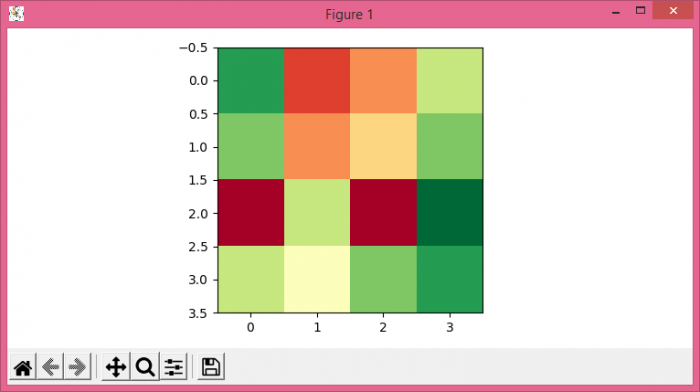# How can I display an np.array with pylab.imshow() using Matplotlib?

To display an np.array with imshow(), we can take the following steps

• Set the figure size and adjust the padding between and around the subplots.
• Make a 2D data raster using an np.array.
• Display the data as an image, i.e., on a 2D regular raster.
• To display the figure, use show() method.

## Example

import numpy as np
from matplotlib import pyplot as plt
plt.rcParams["figure.figsize"] = [7.50, 3.50]
plt.rcParams["figure.autolayout"] = True
data = np.array(
[[0.1, 0.7, 0.6, 0.3],
[0.2, 0.6, 0.5, 0.2],
[0.8, 0.3, 0.80, 0.01],
[0.3, 0.4, 0.2, 0.1]]
)
plt.imshow(data, interpolation="nearest", cmap="RdYlGn_r")
plt.show()

## Output